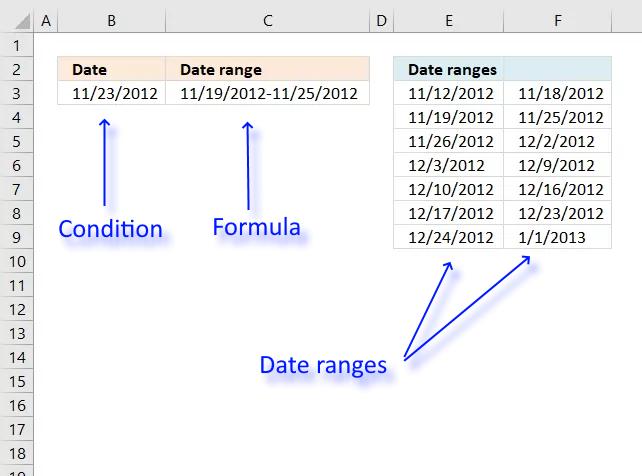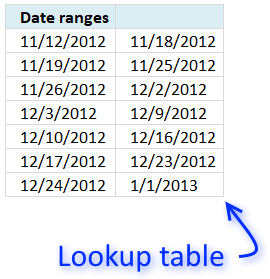Author: Oscar Cronquist Article last updated on October 31, 2019This article demonstrates a formula that returns a date range that a date falls under, cell C3 above contains the formula.

Cell B3 contains the condition and column E and F contain the date ranges.

I need to figure out the date range of a cell. So if cell "E2" has a date of 11/23/2012 then that would need to fall under date range of "11/19 - 11/25".

I have the following date range I need to identify:
"11/12 - 11/18", "11/19 - 11/25", "11/26 - 12/2", "12/3 - 12/9", "12/10 - 12/16", "12/17 - 12/23", "12/24 - 1/1/2013".
I tried the formula below but I keep getting a result of "False".

I appreciate any help I can get. Thank you

The formula in cell C3 returns the correct date range from the lookup table based on the date in cell B3.

Array formula in cell C3:

=TEXT(LOOKUP(B3,E3:E9),"MM/DD/YYYY")&"-"&TEXT(LOOKUP(B3,E3:E9,F3:F9),"MM/DD/YYYY")The lookup table consists of dates beginning with Monday and ends with Sundays.

For example, 11/12/2012 is a Monday and  11/18/2012 is a Sunday.

There are no gaps between the end and start date so the LOOKUP function only needs the first column for it to work.

The following formula calculates the dates if you don't want to use a lookup table.

=TEXT(B3-WEEKDAY(B3,2)+1,"MM/DD/YYYY")&"-"&TEXT(B3-WEEKDAY(B3,2)+7,"MM/DD/YYYY")

This formula works only if the date range starts with a Monday and ends with a Sunday.

Recommended article

Formula for matching a date within a date range

This article demonstrates how to match a specified date to date ranges. The image above shows a formula in cell […]

### Explaining array formula in cell C3

#### Step 1 - Find the matching start date

The LOOKUP function allows you to search for a value and return the largest value that is smaller than the lookup value.

For this to work the table must be sorted from small to large, this is important. You will get unreliable results if this requirement is not met.

Excel dates are actually numbers, 1/1/1900 is 1 and 1/1/2018 is 43101.

Since dates are numbers in Excel you can easily use the LOOKUP function in this case.

becomes

LOOKUP(41236, {41225; 41232; 41239; 41246; 41253; 41260; 41267})

and returns 41232.

41232 is the largest number that is smaller than 41236 in the array.

#### Step 2 - Convert serial number to Excel date

The TEXT function allows you to convert the serial number to a date. The second argument in the TEXT function lets you specify how the date shall look like.

TEXT(LOOKUP(B3,E3:E9),"MM/DD/YYYY")

becomes

TEXT(41232,"MM/DD/YYYY")

and returns 11/19/2012.

#### Step 3 - Find the matching end date

The LOOKUP function lets you also to return a corresponding value on the same row if you enter the third argument in the LOOKUP function.

LOOKUP(B3,E3:E9,F3:F9)

becomes

LOOKUP(41236,{41225; 41232; 41239; 41246; 41253; 41260; 41267},{41231; 41238; 41245; 41252; 41259; 41266; 41275})

and returns 41238 which is 11/25/2012.

The TEXT function converts the serial number to an Excel date, see step 2 again if you need to see the details again.

#### Step 4 - Concatenate the two Excel dates

The & ampersand allows you to concatenate two values in one cell.

TEXT(B3-WEEKDAY(B3,2)+1,"MM/DD/YYYY")&"-"&TEXT(B3-WEEKDAY(B3,2)+7,"MM/DD/YYYY")

becomes

11/19/2012&"-"&11/25/2012

and returns 11/19/2012-11/25/2012 in cell C3.

### Get the Excel fileFind-date-range.xlsx